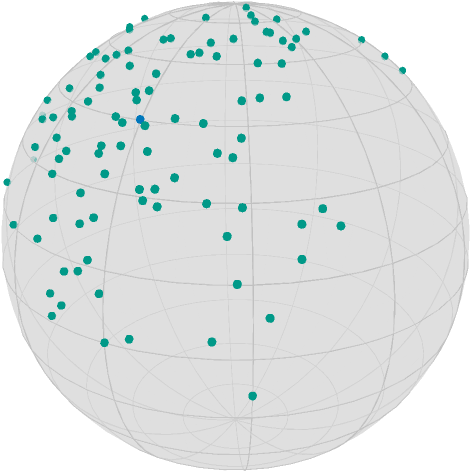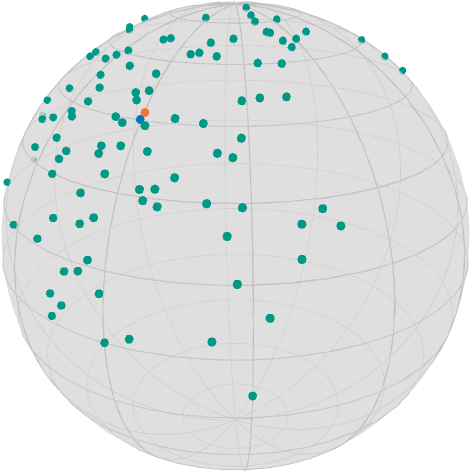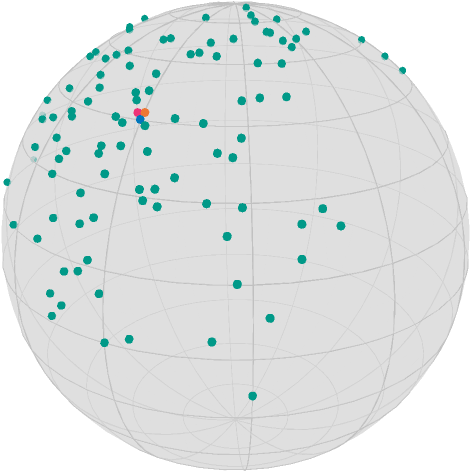# Get started: Optimize!

This example illustrates how to set up and solve optimization problems and how to further get data from the algorithm using debug output and record data. We will use the Riemannian mean and median as simple examples.

To start from the quite general case: A Solver is an algorithm that aims to solve

$$\operatorname*{argmin}_{x∈\mathcal M} f(x)$$

where $\mathcal M$ is a Manifold and $f:\mathcal M → ℝ$ is the cost function.

In Manopt.jl a Solver is an algorithm that requires a Problem p and Options o. While former contains static data, most prominently the manifold $\mathcal M$ (usually as p.M) and the cost function $f$ (usually as x->get_cost(p, x)), the latter contains dynamic data, i.e. things that usually change during the algorithm, are allowed to change, or specify the details of the algorithm to use. Together they form a plan. A plan uniquely determines the algorithm to use and provide all necessary information to run the algorithm.

# Setup

Let‘s first set up a few variables

begin
localpath = join(splitpath(@__FILE__)[1:(end - 1)], "/") # files folder
image_prefix = localpath * "/optimize"
@info image_prefix
render_asy = false # on CI or when you do not have asymptote, this should be false
end;

# Example

A gradient plan consists of a GradientProblem with the fields M, cost function $f$ as well as gradient storing the gradient function corresponding to $f$. Accessing both functions can be done directly but should be encapsulated using get_cost(p,x) and get_gradient(p,x), where in both cases x is a point on the Manifold M. Second, the GradientDescentOptions specify that the algorithm to solve the GradientProblem is the gradient descent algorithm. It requires an initial value o.x0, a StoppingCriterion o.stop, a Stepsize o.stepsize and a retraction o.retraction. Internally is stores the last evaluation of the gradient at o.gradient for convenience. The only mandatory parameter is the initial value x0, though the defaults for both the stopping criterion (StopAfterIteration(100)) as well as the stepsize (ConstantStepsize(1.) are quite conservative, but are chosen to be as simple as possible.

With these two at hand, running the algorithm just requires to call x_opt = solve(p,o).

In the following two examples we will see, how to use a higher level interface that allows to more easily activate for example a debug output or record values during the iterations.

using Manopt, Manifolds, Random, Colors, PlutoUI

Let‘s load a few colors from Paul Tol

begin
black = RGBA{Float64}(colorant"#000000")
TolVibrantOrange = RGBA{Float64}(colorant"#EE7733")
TolVibrantBlue = RGBA{Float64}(colorant"#0077BB")
TolVibrantTeal = RGBA{Float64}(colorant"#009988")
TolVibrantMagenta = RGBA{Float64}(colorant"#EE3377")
end;

# The data set

We take a look at a srandom set of points.

begin
n = 100
σ = π / 8
M = Sphere(2)
x = 1 / sqrt(2) * [1.0, 0.0, 1.0]
Random.seed!(42)
data = [exp(M, x, random_tangent(M, x, Val(:Gaussian), σ)) for i in 1:n]
end
100-element Vector{Vector{Float64}}:
[0.9013817292525768, -0.3266930346539438, 0.2842228690275139]
[0.5413128376734956, -0.27073756886516653, 0.7960411927625647]
[0.11640703550675724, 0.22835279004025902, 0.9665942299462364]
[0.8120710958917794, 0.3048940575972932, 0.4975742646670772]
[0.3403759726989105, 0.16278898828946248, 0.9260906772562628]
[0.7937947657628919, -0.11512297480761367, 0.5971905646599591]
[0.8657115032997766, 0.0994828021701859, 0.49055760632856193]
⋮
[-0.24540230556351394, 0.6192543288278474, 0.7458564102104935]
[0.7206649443671707, 0.026606223795364103, 0.6927727959552205]
[-0.1556170638176626, 0.4709844443817593, 0.8683069633483166]
[0.864645918606244, 0.15287339377544437, 0.47855737473488213]
[0.8283553683993905, 0.539804408097735, 0.14979514225155605]
[0.7766227187182986, -0.11478608022900104, 0.6194201389656778]
asymptote_export_S2_signals(
image_prefix * "/startDataAndCenter.asy";
points=[[x], data],
colors=Dict(:points => [TolVibrantBlue, TolVibrantTeal]),
dot_size=3.5,
camera_position=(1.0, 0.5, 0.5),
)
render_asy && render_asymptote(image_prefix * "/startDataAndCenter.asy"; render=2)
false
PlutoUI.LocalResource(image_prefix * "/startDataAndCenter.png")## Computing the Mean

To compute the mean on the manifold we use the characterization, that the Euclidean mean minimizes the sum of squared distances, and end up with the following cost function. Its minimizer is called Riemannian Center of Mass.

Note. There are more sophisticated methods tailored for the specific manifolds available in Manifolds.jl see mean.

F(M, y) = sum(1 / (2 * n) * distance.(Ref(M), Ref(y), data) .^ 2)
F (generic function with 1 method)
gradF(M, y) = sum(1 / n * grad_distance.(Ref(M), data, Ref(y)))
gradF (generic function with 1 method)

Note that the grad_distance defaults to the case p=2, i.e. the gradient of the squared distance. For details on convergence of the gradient descent for this problem, see [AfsariTronVidal2013]

The easiest way to call the gradient descent is now to call gradient_descent.

gradient_descent(M, F, gradF, data)
3-element Vector{Float64}:
0.6868392769979478
0.006531602662116221
0.7267799844104132

In order to get more details, we further add the debug= options, which act as a decorator pattern.

The following debug prints

# i | Last Change: | F(x): | x:

as well as the reason why the algorithm stopped at the end.

The elements passed to debug= are postprocessed, there are specifiic symbols and formats we can use. For example :Iteration to plot the iteratin, but we can also add a format for the print using (:Change, "| Last change: %e3.8"), i.e. the second string is a format for printf.

Note that here we use PlutoUI to see the output also here in the notebook

with_terminal() do
M,
F,
data;
debug=[
:Iteration,
(:Change, "change: %1.9f | "),
(:Cost, " F(x): %1.11f | "),
"\n",
:Stop,
],
)
end
Initial  F(x): 0.32487988924 |
# 1     change: 0.902786697 |  F(x): 0.19419821689 |
# 2     change: 0.447944200 |  F(x): 0.16194066921 |
# 3     change: 0.222196863 |  F(x): 0.15405087889 |
# 4     change: 0.110584791 |  F(x): 0.15209325188 |
# 5     change: 0.054993453 |  F(x): 0.15160970742 |
# 6     change: 0.027365893 |  F(x): 0.15148991364 |
# 7     change: 0.013615009 |  F(x): 0.15146027110 |
# 8     change: 0.006774919 |  F(x): 0.15145293057 |
# 9     change: 0.003371155 |  F(x): 0.15145111325 |
# 10    change: 0.001677580 |  F(x): 0.15145066322 |
# 11    change: 0.000834825 |  F(x): 0.15145055178 |
# 12    change: 0.000415455 |  F(x): 0.15145052418 |
# 13    change: 0.000206759 |  F(x): 0.15145051734 |
# 14    change: 0.000102900 |  F(x): 0.15145051565 |
# 15    change: 0.000051213 |  F(x): 0.15145051523 |
# 16    change: 0.000025489 |  F(x): 0.15145051513 |
# 17    change: 0.000012686 |  F(x): 0.15145051510 |
# 18    change: 0.000006314 |  F(x): 0.15145051509 |
# 19    change: 0.000003143 |  F(x): 0.15145051509 |
# 20    change: 0.000001564 |  F(x): 0.15145051509 |
# 21    change: 0.000000779 |  F(x): 0.15145051509 |
# 22    change: 0.000000387 |  F(x): 0.15145051509 |
# 23    change: 0.000000191 |  F(x): 0.15145051509 |
# 24    change: 0.000000094 |  F(x): 0.15145051509 |
# 25    change: 0.000000045 |  F(x): 0.15145051509 |
# 26    change: 0.000000021 |  F(x): 0.15145051509 |
# 27    change: 0.000000015 |  F(x): 0.15145051509 |
The algorithm reached approximately critical point after 27 iterations; the gradient norm (7.540089513196966e-9) is less than 1.0e-8.


A way to get better performance and for convex and coercive costs a guaranteed convergence is to switch the default ConstantStepsize(1.0) with a step size that performs better, for example the ArmijoLinesearch. We can tweak the default values for the contraction_factor and the sufficient_decrease beyond constant step size which is already quite fast. We get

with_terminal() do
M,
F,
data;
stepsize=ArmijoLinesearch(1.0, ExponentialRetraction(), 0.99, 0.5),
debug=[
:Iteration, (:Change, "change: %1.9f | "), :Cost, (:x, " | %s"), "\n", :Stop
],
)
end
Initial F(x): 0.324880 | [0.9013817292525768, -0.3266930346539438, 0.2842228690275139]
# 1     change: 0.598307567 | F(x): 0.151463 | [0.6891648805180924, 0.0026275812635667695, 0.7245997952505829]
# 2     change: 0.004992928 | F(x): 0.151451 | [0.6868477184189742, 0.006479791589843487, 0.7267724705873233]
# 3     change: 0.000052757 | F(x): 0.151451 | [0.6868390793259461, 0.0065312628686383335, 0.7267801742728178]
# 4     change: 0.000000433 | F(x): 0.151451 | [0.6868392781604383, 0.006531596591309469, 0.7267799833663667]
# 5     change: 0.000000015 | F(x): 0.151451 | [0.6868392794629642, 0.00653160063944062, 0.7267799820990414]
The algorithm reached approximately critical point after 5 iterations; the gradient norm (4.2198733567896755e-9) is less than 1.0e-8.


which finishes in 5 steaps, just slightly better than the previous computation.

F(M, xMean) - F(M, xMean2)
2.7755575615628914e-17

Note that other optimization tasks may have other speedup opportunities.

For even more precision, we can further require a smaller gradient norm. This is done by changing the StoppingCriterion used, where several criteria can be combined using & and/or |. If we want to decrease the final gradient (from less that 1e-8) norm but keep the maximal number of iterations to be 200, we can run

with_terminal() do
M,
F,
data;
stepsize=ArmijoLinesearch(1.0, ExponentialRetraction(), 0.99, 0.5),
debug=[
:Iteration,
(:Change, "change: %1.9f | "),
(:Cost, "F(x): %1.9f"),
(:x, " | %s"),
"\n",
:Stop,
],
)
end
Initial F(x): 0.324879889 | [0.9013817292525768, -0.3266930346539438, 0.2842228690275139]
# 1     change: 0.598307567 | F(x): 0.151462602 | [0.6891648805180924, 0.0026275812635667695, 0.7245997952505829]
# 2     change: 0.004992928 | F(x): 0.151450516 | [0.6868477184189742, 0.006479791589843487, 0.7267724705873233]
# 3     change: 0.000052757 | F(x): 0.151450515 | [0.6868390793259461, 0.0065312628686383335, 0.7267801742728178]
# 4     change: 0.000000433 | F(x): 0.151450515 | [0.6868392781604383, 0.006531596591309469, 0.7267799833663667]
# 5     change: 0.000000015 | F(x): 0.151450515 | [0.6868392794629642, 0.00653160063944062, 0.7267799820990414]
# 6     change: 0.000000015 | F(x): 0.151450515 | [0.6868392794779344, 0.006531600678337795, 0.7267799820845443]
# 7     change: 0.000000015 | F(x): 0.151450515 | [0.6868392794787861, 0.006531600680566919, 0.7267799820837194]
# 8     change: 0.000000000 | F(x): 0.151450515 | [0.6868392794788591, 0.006531600680758874, 0.7267799820836487]
# 9     change: 0.000000000 | F(x): 0.151450515 | [0.6868392794788661, 0.006531600680777358, 0.7267799820836419]
# 10    change: 0.000000000 | F(x): 0.151450515 | [0.6868392794788668, 0.006531600680779006, 0.7267799820836414]
# 11    change: 0.000000000 | F(x): 0.151450515 | [0.6868392794788669, 0.006531600680779264, 0.7267799820836413]
The algorithm reached approximately critical point after 11 iterations; the gradient norm (3.1145754488467843e-16) is less than 1.0e-15.


Let‘s add this point to out data image

asymptote_export_S2_signals(
image_prefix * "/startDataCenterMean.asy";
points=[[x], data, [xMean3]],
colors=Dict(:points => [TolVibrantBlue, TolVibrantTeal, TolVibrantOrange]),
dot_size=3.5,
camera_position=(1.0, 0.5, 0.5),
)
render_asy && render_asymptote(image_prefix * "/startDataCenterMean.asy"; render=2)
false
PlutoUI.LocalResource(image_prefix * "/startDataCenterMean.png")## Computing the Median

Note. There are more sophisticated methods tailored for the specific manifolds available in Manifolds.jl see median.

Similar to the mean you can also define the median as the minimizer of the distances, see for example [Bačák2014], but since this problem is not differentiable, we employ the Cyclic Proximal Point (CPP) algorithm, described in the same reference. We define

F2(M, y) = sum(1 / (2 * n) * distance.(Ref(M), Ref(y), data))
F2 (generic function with 1 method)
proxes = Function[(M, λ, y) -> prox_distance(M, λ / n, di, y, 1) for di in data]
100-element Vector{Function}:
#11 (generic function with 1 method)
#11 (generic function with 1 method)
#11 (generic function with 1 method)
#11 (generic function with 1 method)
#11 (generic function with 1 method)
#11 (generic function with 1 method)
#11 (generic function with 1 method)
⋮
#11 (generic function with 1 method)
#11 (generic function with 1 method)
#11 (generic function with 1 method)
#11 (generic function with 1 method)
#11 (generic function with 1 method)
#11 (generic function with 1 method)

So we call the cyclic proximal point algorithm this time with a recording and activate the return of the complete options to access the recorded values. We further increase the display of the cost function to more digits.

with_terminal() do
global o = cyclic_proximal_point(
M,
F2,
proxes,
data;
debug=[
:Iteration,
" | ",
:Change,
" | ",
(:Cost, "F(x): %1.12f"),
" | ",
:x,
"\n",
50,
:Stop,
],
record=[:Iteration, :Change, :Cost, :x],
return_options=true,
)
end
Initial  |  | F(x): 0.362208118208 | x: [0.9013817292525768, -0.3266930346539438, 0.2842228690275139]
# 50     | Last Change: 0.134517 | F(x): 0.244370141855 | x: [0.6899935005916552, -0.018961773170945766, 0.7235671498205912]
# 100    | Last Change: 0.000738 | F(x): 0.244369477428 | x: [0.6896989553827804, -0.01962006271968173, 0.7238303696880815]
# 150    | Last Change: 0.000249 | F(x): 0.244369347858 | x: [0.689627709392341, -0.019858554704607612, 0.7238917462185616]
# 200    | Last Change: 0.000125 | F(x): 0.244369301328 | x: [0.6895992327648918, -0.019981699583130192, 0.7239154852963935]
# 250    | Last Change: 0.000075 | F(x): 0.244369279442 | x: [0.6895849904297638, -0.020056887244235287, 0.7239269730077794]
# 300    | Last Change: 0.000050 | F(x): 0.244369267418 | x: [0.6895768884180585, -0.02010756733653098, 0.7239332846993344]
# 350    | Last Change: 0.000036 | F(x): 0.244369260105 | x: [0.6895718777269852, -0.020144043032678104, 0.7239370435185869]
# 400    | Last Change: 0.000027 | F(x): 0.244369255326 | x: [0.6895685928055626, -0.02017155169244206, 0.7239394065241377]
# 450    | Last Change: 0.000021 | F(x): 0.244369252032 | x: [0.689566345525191, -0.02019303761674476, 0.72394094811034]
The algorithm performed a step with a change (0.0) less than 1.0e-12.

xMedian = get_solver_result(o)
3-element Vector{Float64}:
0.6895661616463945
-0.020194930269146295
0.723941070463446

where the differences to gradient_descent are as follows

• the third parameter is now an Array of proximal maps

• debug is reduces to only every 50th iteration

• we further activated a RecordAction using the record= optional parameter. These work very similar to those in debug, but they collect their data in an array. The high level interface then returns two variables; the values do contain an array of recorded datum per iteration. Here a Tuple containing the iteration, last change and cost respectively; see RedordOptions for details.

We can access the recorded values using get_record and contains of a tuple per iteration and contains the iteration number, the change and the cost.

values = get_record(o)
455-element Vector{Tuple{Int64, Float64, Float64, Vector{Float64}}}:
(1, 0.0, 0.25122077492914485, [0.7725364607817969, -0.07080234064197369, 0.6310106538897339])
(2, 0.07946683931821867, 0.24559809471687633, [0.7299963552108888, -0.028225181418871072, 0.6828679671156679])
(3, 0.025458747868814337, 0.24476563892261735, [0.7136354125490967, -0.019375250667647812, 0.7002493110438961])
(4, 0.011128576957056035, 0.2445520584148201, [0.7060405381827376, -0.016820341944346014, 0.7079716339935571])
(5, 0.0060558247322904735, 0.24447264231227728, [0.7018014581124855, -0.01589109362362358, 0.7121953289194127])
(6, 0.0037583754395689806, 0.24443554267519946, [0.6991317792895255, -0.015584930889514396, 0.7148229606809152])
(7, 0.0025374768125748263, 0.24441546613892315, [0.6973159854821999, -0.015551439967875317, 0.7165951221616729])
⋮
(450, 1.794337418495463e-7, 0.24436925203177753, [0.689566345525191, -0.02019303761674476, 0.72394094811034])
(451, 1.7120130796843874e-7, 0.2443692519766887, [0.6895663082401093, -0.0201934194553006, 0.7239409729742272])
(452, 1.5269146510898484e-7, 0.2443692519219647, [0.6895662712118454, -0.020193799629004104, 0.7239409976397223])
(453, 1.1542389828584848e-7, 0.24436925186759018, [0.6895662344382355, -0.020194178148716987, 0.7239410221085976])
(454, 3.942476676500724e-8, 0.24436925181357566, [0.6895661979171256, -0.020194555025206357, 0.7239410463825942])
(455, 0.0, 0.2443692517599089, [0.6895661616463945, -0.020194930269146295, 0.723941070463446])
asymptote_export_S2_signals(
image_prefix * "/startDataCenterMedianAndMean.asy";
points=[[x], data, [xMean], [xMedian]],
colors=Dict(
:points => [TolVibrantBlue, TolVibrantTeal, TolVibrantOrange, TolVibrantMagenta]
),
dot_size=3.5,
camera_position=(1.0, 0.5, 0.5),
)
render_asy && render_asymptote(image_prefix * "/startDataCenterMedianAndMean.asy"; render=2)
false

In the following image the mean (orange), median (magenta) are shown.

PlutoUI.LocalResource(image_prefix * "/startDataCenterMedianAndMean.png")## Literature

Bačák2014

Bačák, M: Computing Medians and Means in Hadamard Spaces. SIAM Journal on Optimization, Volume 24, Number 3, pp. 1542–1566, doi: 10.1137/140953393, arxiv: 1210.2145

AfsariTronVidal2013

Afsari, B; Tron, R.; Vidal, R.: On the Convergence of Gradient Descent for Finding the Riemannian Center of Mass, SIAM Journal on Control and Optimization, Volume 51, Issue 3, pp. 2230–2260. doi: 10.1137/12086282X, arxiv: 1201.0925.